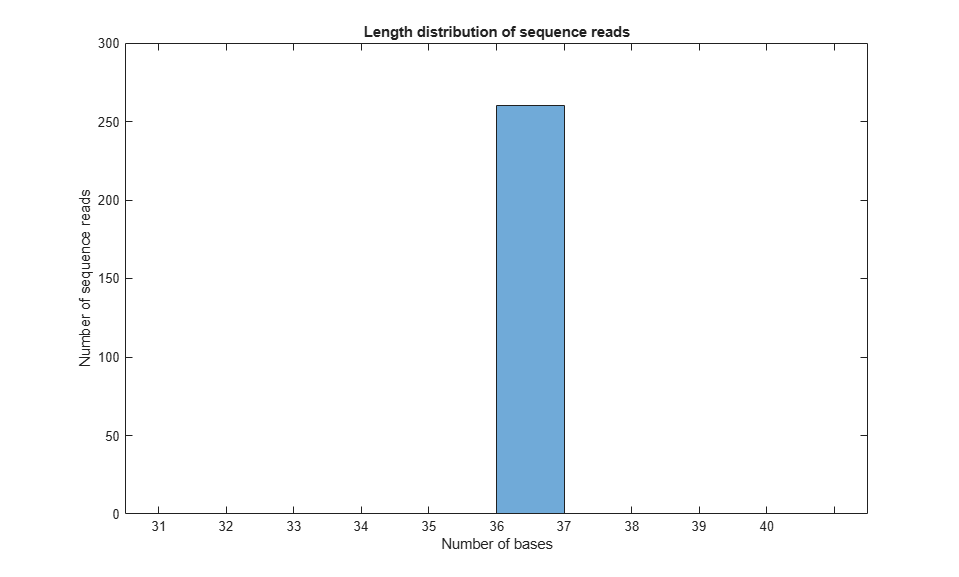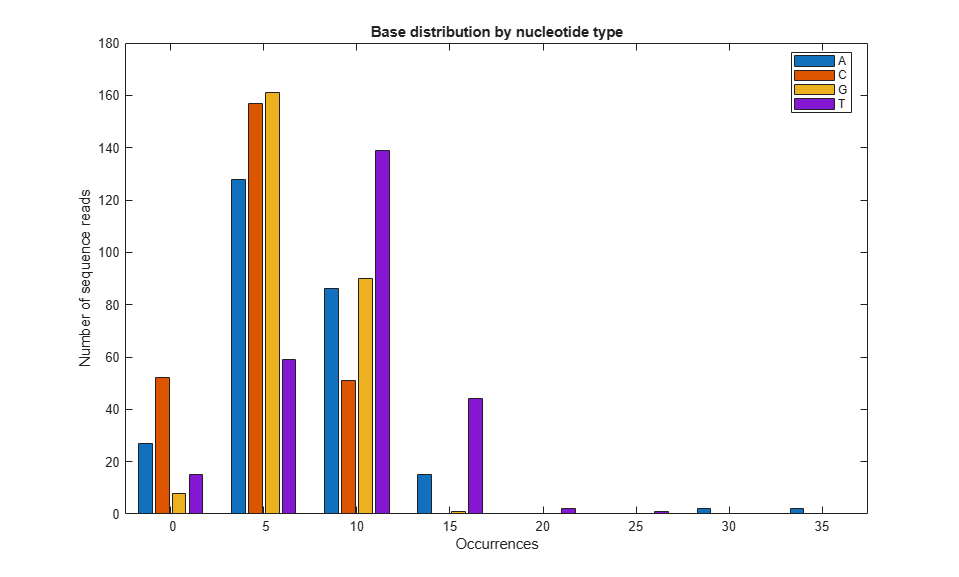# Working with Illumina®/Solexa Next-Generation Sequencing Data

This example shows how to read and perform basic operations with data produced by the Illumina/Solexa Genome Analyzer®.

### Introduction

During an analysis run with the Genome Analyzer Pipeline software, several intermediate files are produced. In this example, you will learn how to read and manipulate the information contained in sequence files (`_sequence.txt`).

The `_sequence.txt` files are FASTQ-formatted files that contain the sequence reads and their quality scores, after quality trimming and filtering. You can use the `fastqinfo` function to display a summary of the contents of a `_sequence.txt` file, and the `fastqread` function to read the contents of the file. The output, `reads`, is a cell array of structures containing the `Header`, `Sequence` and `Quality` fields.

```filename = 'ilmnsolexa_sequence.txt'; info = fastqinfo(filename) reads = fastqread(filename) ```
```info = struct with fields: Filename: 'ilmnsolexa_sequence.txt' FilePath: '/tmp/Bdoc21a_1606923_70404/tp8a08b52d/ex25447385' FileModDate: '06-May-2009 16:02:48' FileSize: 30124 NumberOfEntries: 260 reads = 1x260 struct array with fields: Header Sequence Quality ```

Because there is one sequence file per tile, it is not uncommon to have a collection of over 1,000 files in total. You can read the entire collection of files associated with a given analysis run by concatenating the _sequence.txt files into a single file. However, because this operation usually produces a large file that requires ample memory to be stored and processed, it is advisable to read the content in chunks using the `blockread` option of the `fastqread` function. For example, you can read the first M sequences, or the last M sequences, or any M sequences in the file.

```M = 150; N = info.NumberOfEntries; readsFirst = fastqread(filename, 'blockread', [1 M]) readsLast = fastqread(filename, 'blockread', [N-M+1, N]) ```
```readsFirst = 1x150 struct array with fields: Header Sequence Quality readsLast = 1x150 struct array with fields: Header Sequence Quality ```

### Surveying the Length Distribution of Sequence Reads

Once you load the sequence information into your workspace, you can determine the number and length of the sequence reads and plot their distribution as follows:

```seqs = {reads.Sequence}; readsLen = cellfun(@length, seqs); figure(); hist(readsLen); xlabel('Number of bases'); ylabel('Number of sequence reads'); title('Length distribution of sequence reads') ```As expected, in this example all sequence reads are 36 bp long.

### Surveying the Base Composition of the Sequence Reads

You can also examine the nucleotide composition by surveying the number of occurrences of each base type in each sequence read, as shown below:

```nt = {'A', 'C', 'G', 'T'}; pos = cell(4,N); for i = 1:4 pos(i,:) = strfind(seqs, nt{i}); end count = zeros(4,N); for i = 1:4 count(i,:) = cellfun(@length, pos(i,:)); end %=== plot nucleotide distribution figure(); subplot(2,2,1); hist(count(1,:)); title('A'); ylabel('Number of sequence reads'); subplot(2,2,2); hist(count(2,:)); title('C'); subplot(2,2,3); hist(count(3,:)); title('G'); xlabel('Occurrences'); ylabel('Number of sequence reads'); subplot(2,2,4); hist(count(4,:)); title('T'); xlabel('Occurrences'); figure(); hist(count'); xlabel('Occurrences'); ylabel('Number of sequence reads'); legend('A', 'C', 'G', 'T'); title('Base distribution by nucleotide type'); ```### Surveying the Quality Score Distribution

Each sequence read in the `_sequence.txt` file is associated with a score. The score is defined as SQ = -10 * log10 (p / (1-p)), where p is the probability error of a base. You can examine the quality scores associated with the base calls by converting the ASCII format into a numeric representation, and then plotting their distribution, as shown below:

```sq = {reads.Quality}; % in ASCII format SQ = cellfun(@(x) double(x)-64, {reads.Quality}, 'UniformOutput', false); % in integer format %=== average, median and standard deviation avgSQ = cellfun(@mean, SQ); medSQ = cellfun(@median, SQ); stdSQ = cellfun(@std, SQ); %=== plot distribution of median and average quality figure(); subplot(1,2,1); hist(medSQ); xlabel('Median Score SQ'); ylabel('Number of sequence reads'); subplot(1,2,2); boxplot(avgSQ); ylabel('Average Score SQ'); ```### Converting Quality Scores Between Standards

The quality scores found in Solexa/Illumina files are asymptotic, but not identical, to the quality scores used in the Sanger standard (Phred-like scores, Q). Q is defined as -10 * log10 (p), where p is the error probability of a base. For example, if the quality score of a base is Q = 20, then p = 10 ^(-20/10) = .01. This means that there is one wrong base call every 100 base calls with a score of20.

While Phred quality scores are positive integers, Solexa/Illumina quality scores can be negative. We can convert Solexa quality scores into Phred quality scores using the following code:

```%=== convert from Solexa to Sanger standard Q = cellfun(@(x) floor(.499 + 10 * log10(1+ 10 .^ (x/10))), SQ, ... 'UniformOutput', false); % in integer format q = cellfun(@(x) char(x+33), Q, 'UniformOutput', false); % in ascii format sanger = q(1:3)' solexa = sq(1:3)' ```
```sanger = 3x1 cell array {'>>>>>>>>>>>>:>:>>>>>>>>>>>>7&*7.1-%4'} {'>>>>>>>>>>>>:>>>>>>>>>:17>5><1;1+&&,'} {'>>>>:>>>>>7>5>>>>>5>>>>>7>5.+'69'(-%'} solexa = 3x1 cell array {']]]]]]]]]]]]Y]Y]]]]]]]]]]]]VCHVMPLAS'} {']]]]]]]]]]]]Y]]]]]]]]]YPV]T][PZPICCK'} {']]]]Y]]]]]V]T]]]]]T]]]]]V]TMJEUXEFLA'} ```

### Filtering and Masking According to Quality Scores

Signal purity filtering has already been applied to the sequences in the `_sequence.txt` files. You can perform additional filtering, for example by considering only those sequence reads whose bases have all quality scores above a specific threshold:

```%=== find sequence reads whose bases all have quality above threshold len = 36; qt = 10; % minimum quality threshold a = cellfun(@(x) x > qt, SQ, 'UniformOutput', false); b = cellfun(@sum, a); c1 = find(b == len); n1= numel(c1); % number of sequence reads passing the filter disp([num2str(n1) ' sequence reads have all bases above threshold ' num2str(qt)]); ```
```30 sequence reads have all bases above threshold 10 ```

Alternatively, you can consider only those sequence reads that have less than a given number of bases with quality scores below threshold:

```%=== find sequence reads having less than M bases with quality below threshold M = 5; % max number of bases with poor quality a = cellfun(@(x) x <= qt, SQ, 'UniformOutput', false); b = cellfun(@sum, a); c2 = find(b <= M); n2 = numel(c2); % number of sequence reads passing the filter disp([num2str(n2) ' sequence reads have less than ' num2str(M) ' bases below threshold ' num2str(qt)]); ```
```235 sequence reads have less than 5 bases below threshold 10 ```

Finally, you can apply a lower case mask to those bases that have quality scores below threshold:

```seq = reads(1).Sequence mseq = seq; qt2 = 20; % quality threshold mask = SQ{1} < qt; mseq(mask) = lower(seq(mask)) ```
```seq = 'GGACTTTGTAGGATACCCTCGCTTTCCTTCTCCTGT' mseq = 'GGACTTTGTAGGATACCCTCGCTTTCCTtcTCCTgT' ```

To summarize read occurrences, you can determine the number of unique read sequences and their distribution across the data set. You can also identify those sequence reads that occur multiple times, often because they correspond to adapters or primers used in the sequencing process.

```%=== determine read frequency [uReads,~,n] = unique({reads.Sequence}); numUnique = numel(uReads) readFreq = accumarray(n(:),1); figure(); hist(readFreq, unique(readFreq)); xlabel('Occurrences'); ylabel('Number of sequence reads'); title('Read occurrences'); %=== identify multiply-occurring sequence reads d = readFreq > 1; dupReads = uReads(d)' dupFreq = readFreq(d)' ```
```numUnique = 250 dupReads = 9x1 cell array {'AAAAAAAAAAAAAAAAAAAAAAAAAAAAAAAAAAAA'} {'GATTTTATTGGTATCAGGGTTAATCGTGCCAAGAAA'} {'GCATGGGTGATGCTGGTATTAAATCTGCCATTCAAG'} {'GGGATGAACATAATAAGCAATGACGGCAGCAATAAA'} {'GGGGGAGCACATTGTAGCATTGTGCCAATTCATCCA'} {'GGTTATTAAAGAGATTATTTGTCTCCAGCCACTTAA'} {'GTTCTCACTTCTGTTACTCCAGCTTCTTCGGCACCT'} {'GTTGCTGCCATCTCAAAAACATTTGGACTGCTCCGC'} {'GTTGGTTTCTATGTGGCTAAATACGTTAACAAAAAG'} dupFreq = 2 2 2 2 2 2 3 2 2 ```### Identifying Homopolymers Artifacts

Illumina/Solexa sequencing may produce false polyA at the edges of a tile. To identify these artifacts, you need to identify homopolymers, that is, sequence reads composed of one type of nucleotide only. In the data set under consideration, there are two homopolymers, both of which are polyA.

```%=== find homopolymers pc = (count ./ len) * 100; [homopolType,homopolIndex] = find(pc == 100); homopolIndex homopol = {reads(homopolIndex).Sequence}' ```
```homopolIndex = 251 257 homopol = 2x1 cell array {'AAAAAAAAAAAAAAAAAAAAAAAAAAAAAAAAAAAA'} {'AAAAAAAAAAAAAAAAAAAAAAAAAAAAAAAAAAAA'} ```

Similarly, you can identify sequence reads that are near-matches to homopolymers, that is, sequence reads that are composed almost exclusively of one nucleotide type.

```%=== find near-homopolymers [nearhomopolType, nearhomopolIndex] = find(pc < 100 & pc > 85); % more than 85% same base nearhomopolIndex nearHomopol = {reads(nearhomopolIndex).Sequence}' ```
```nearhomopolIndex = 4 243 nearHomopol = 2x1 cell array {'AAAAACATAAAAAAAAAAATAAAAAAACAAAAAAAA'} {'AAAAAAATAAAAAAAAAAATAAAAAAAAATTAAAAA'} ```

### Writing Data to FASTQ Format

Once you have processed and analyzed your data, it might be convenient to save a subset of sequences in a separate FASTQ file for future consideration. For this purpose you can use the `fastqwrite` function.

## SupportGet trial now